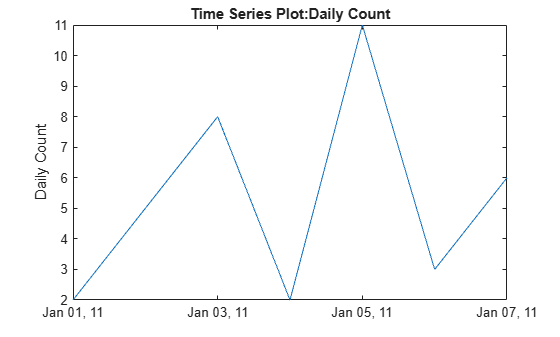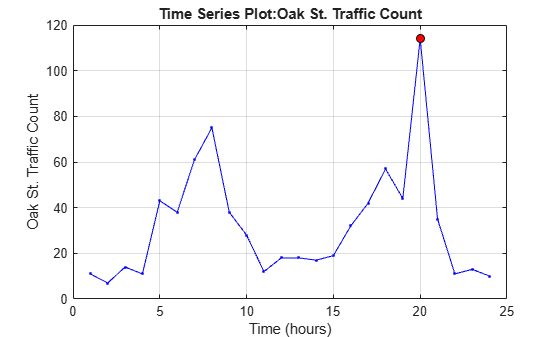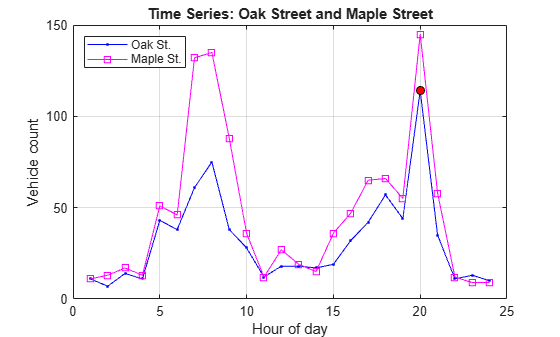# plot

Plot `timeseries`

## Syntax

``plot(ts)``
``plot(ts,LineSpec)``

## Description

example

````plot(ts)` plots the `timeseries` data in `ts` against time, interpolating values between samples.```

example

````plot(ts,LineSpec)` plots the `timeseries` data using a line graph and applies the specified `specs` to lines, markers, or both.You can also specify name-value arguments to define Line Properties.```

## Examples

collapse all

Create a time series object, set the start date, and then plot the time vector relative to the start date.

```x = [2 5 8 2 11 3 6]; ts1 = timeseries(x,1:7); ts1.Name = 'Daily Count'; ts1.TimeInfo.Units = 'days'; ts1.TimeInfo.StartDate = '01-Jan-2011'; % Set start date. ts1.TimeInfo.Format = 'mmm dd, yy'; % Set format for display on x-axis. ts1.Time = ts1.Time - ts1.Time(1); % Express time relative to the start date. plot(ts1)```Create two time series objects from traffic count data, and then plot them in sequence on the same axes. Add an event to one series, which is automatically displayed with a red marker.

```load count.dat; count1 = timeseries(count(:,1),1:24); count1.Name = 'Oak St. Traffic Count'; count1.TimeInfo.Units = 'hours'; plot(count1,':b') grid on```Obtain time of maximum value and add it as an event:

```[~,index] = max(count1.Data); max_event = tsdata.event('peak',count1.Time(index)); max_event.Units = 'hours';```

Add the event to the time series:

`count1 = addevent(count1,max_event);`

Replace plot with new one showing the event:

```plot(count1,'.-b') grid on```Make a new time series object from column 2 of the same data source:

```count2 = timeseries(count(:,2),1:24); count2.Name = 'Maple St. Traffic Count'; count2.TimeInfo.Units = 'Hours';```

Turn hold on to add the new data to the plot:

`hold on`

The plot method does not add labels to a held plot. Use property/value pairs to customize markers:

`plot(count2,'s-m','MarkerSize',6),`Labels are erased, so generate them manually:

```title('Time Series: Oak Street and Maple Street') xlabel('Hour of day') ylabel('Vehicle count')```

Add a legend in the upper left:

`legend('Oak St.','Maple St.','Location','northwest')`## Input Arguments

collapse all

Input `timeseries`, specified as a scalar.

Line style, marker, and color, specified as a character vector or string containing symbols. The symbols can appear in any order. You do not need to specify all three characteristics (line style, marker, and color). For example, if you omit the line style and specify the marker, then the plot shows only the marker and no line.

Example: `'--or'` is a red dashed line with circle markers

Line StyleDescriptionResulting Line
`'-'`Solid line`'--'`Dashed line`':'`Dotted line`'-.'`Dash-dotted lineMarkerDescriptionResulting Marker
`'o'`Circle`'+'`Plus sign`'*'`Asterisk`'.'`Point`'x'`Cross`'_'`Horizontal line`'|'`Vertical line`'s'`Square`'d'`Diamond`'^'`Upward-pointing triangle`'v'`Downward-pointing triangle`'>'`Right-pointing triangle`'<'`Left-pointing triangle`'p'`Pentagram`'h'`HexagramColor NameShort NameRGB TripletAppearance
`'red'``'r'``[1 0 0]``'green'``'g'``[0 1 0]``'blue'``'b'``[0 0 1]``'cyan'` `'c'``[0 1 1]``'magenta'``'m'``[1 0 1]``'yellow'``'y'``[1 1 0]``'black'``'k'``[0 0 0]``'white'``'w'``[1 1 1]`## Tips

• The `plot` function generates titles and axis labels automatically. These labels are:

• Plot Title — ```'Time Series Plot: <name>'```

where `<name>` is the string assigned to `ts.Name`, or by default, `'unnamed'`

• X-Axis Label — ```'Time (<units>)'```

where `<units>` is the value of the `ts.TimeInfo.Units` field, which defaults to `'seconds'`

• Y-Axis Label — `'<name>'`

where `<name>` is the string assigned to `ts.Name`, or by default, `'unnamed'`

• You can place new time series data on a time series plot (by setting `hold on`, for example, and issuing another `timeseries/plot` command). When you add data to a plot, the title and axis labels become blank strings to avoid labeling confusion. You can add your own labels after plotting using the `title`, `xlabel`, and `ylabel` commands.

• Time series events, when defined, are marked in the plot with a circular marker with red fill. You can also specify markers for all data points using a `linespec` or name/value syntax in addition to any event markers your data defines. The event markers plot on top of the markers you define.

• The value assigned to `ts.DataInfo.Interpolation.Name` controls the type of interpolation the `plot` method uses when plotting and resampling time series data. Invoke the `timeseries` method `setinterpmethod` to change default linear interpolation to zero-order hold interpolation (staircase). This method creates a new `timeseries` object, with which you can overwrite the original one if you want. For example, to cause time series `ts` to use zero-order hold interpolation, type the following:

`ts = ts.setinterpmethod('zoh');`

## Version History

Introduced before R2006a